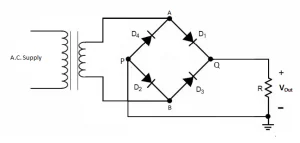# What is Bridge Rectifier? – Circuit Diagram, Working

A rectifier is an electrical circuit that turns an alternating current voltage input into a direct current voltage at the output terminal. The rectified output voltage is the name given to this output. Rectifiers are primarily used in power supplies, supplying DC voltage to electronic equipment.

Rectifiers are classified into two categories based on their operation-

• Half Wave Rectifiers
• Full Wave Rectifiers

Full Wave Rectifiers have been more effective than Half Wave Rectifiers in increasing the efficiency of the rectification. Full Wave Rectifiers generate an output voltage by using both positive and negative half cycles of the input voltage.

Full wave rectifiers are of two types:

• Bridge Full Wave Rectifier
• Centre Tap Full Wave Rectifier

## What is Bridge Rectifier?

Bridge Rectifiers are Full Wave Rectifiers that use four diodes to create a close-loop bridge. The diodes operate in pairs during each positive and negative half cycle, resulting in no power waste.

A bridge rectifier does not require a center tap over the transformer’s secondary winding. The input is sent through a transformer to the diode bridge’s diagonal. Unlike the center tap rectifier, which consumes 50% of the transformer, the transformer in this circuit is constantly busy since it delivers power during both cycles of input AC.

## Bridge Rectifier Circuit Diagram## Bridge Rectifier Waveform## Working of Bridge Rectifier

When an AC is passed through the full wave bridge rectifier, terminal A becomes positive in the positive half cycle, and terminal B becomes negative in the negative half cycle. As a result, diodes D1 and D3 become forward-biased, whereas diodes D2 and D4 become reverse-biased.

During the negative half-cycle, the voltage at terminal B becomes positive while the voltage at terminal A becomes negative. This leads diodes D2 and D4 to be forward-biased while diodes D1 and D3 remain reverse-biased.

As a result, a bridge rectifier allows electric current to flow during both the positive and negative half cycles of the input AC.

## Bridge Rectifier Formulae

These are some characteristics of the full wave bridge rectifier

#### Ripple Factor

The ripple factor is a factor that measures the smoothness of the output DC signal. The output DC with more occasional ripples is referred to as a smooth DC signal, whereas if the output is with more ripples, it is a high-pulsating DC signal.

The ripple factor mathematically is defined as the ratio of ripple voltage to pure DC voltage.

A bridge rectifier’s ripple factor is given by

$$\gamma = \sqrt{ (\frac{ V_{rms} }{ V_{dc} }) ^{2}-1}$$

The ripple factor for bridge rectifiers is 0.48.

#### Peak Inverse Voltage

A peak inverse voltage is the greatest voltage that a diode can endure when biased in reverse. The diodes D1 and D3 are conducted during the positive half cycle, whereas D2 and D4 are not. Similarly, during the negative half cycle, diodes D2 and D4 current whereas diodes D1 and D3 do not.

#### Efficiency

The efficiency of a rectifier influences how well it converts Alternating Current (AC) into Direct Current (DC). A bridge rectifier’s highest efficiency is 81.2%.

$$\eta = \frac{DC Output Power}{AC OutputPower}$$

## Pros and Cons of Bridge Rectifier

#### Advantages of Full Wave Bridge Rectifier

• The efficiency of a bridge rectifier is greater than that of a half-wave rectifier. The rectifier efficiency of the bridge rectifier and the center-tapped full-wave rectifier, on the other hand, is the same.
• The bridge rectifier’s DC output signal is smoother than the DC output signal of a half-wave rectifier.
• A half-wave rectifier uses just half of the incoming AC signal and blocks the other half. A half-wave rectifier wastes half of the input signal. A bridge rectifier lets electricity flow through both the positive and negative halves. As a result, the output DC signal is almost equivalent to the input AC signal.

• A bridge rectifier’s circuit is more complicated than that of a half-wave rectifier or a center-tapped full-wave rectifier. Bridge rectifiers require four diodes, whereas half-wave and center-tapped full-wave rectifiers need just two.
• As more diodes are utilized, more power is lost. Only one diode is conducted during each half cycle of a center-tapped full-wave rectifier. With a bridge rectifier, on the other hand, two diodes linked in series conduct throughout each half cycle. As a result, the voltage drop is larger with a bridge rectifier.

## Final Notes

RMS Voltage = $$V_{rms}= \frac{ V_{m} }{ \sqrt{2} }$$